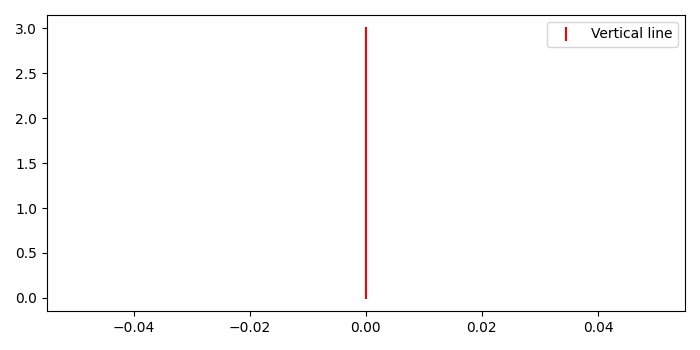# Legend with vertical line in matplotlib

To add a legend with vertical line in matplotlib, we can take the following steps −

• Set the figure size and adjust the padding between and around the subplots.
• Create a figure and a set of subplots.
• Plot the vertical line with red color.
• The line can have both a solid linestyle connecting all the vertices, and a marker at each vertex.
• Place a legend on the plot with vertical line.
• To display the figure, use show() method.

## Example

import matplotlib.pyplot as plt
from matplotlib import lines

plt.rcParams["figure.figsize"] = [7.00, 3.50]
plt.rcParams["figure.autolayout"] = True

fig, ax = plt.subplots()

color = 'red'
ax.plot([0, 0], [0, 3], color=color)

vertical_line = lines.Line2D([], [], color=color, marker='|',
linestyle='None', markersize=10,
markeredgewidth=1.5, label='Vertical line')

plt.legend(handles=[vertical_line], loc='upper right')

plt.show()

## Output

It will produce the following output